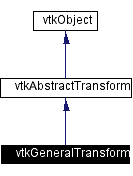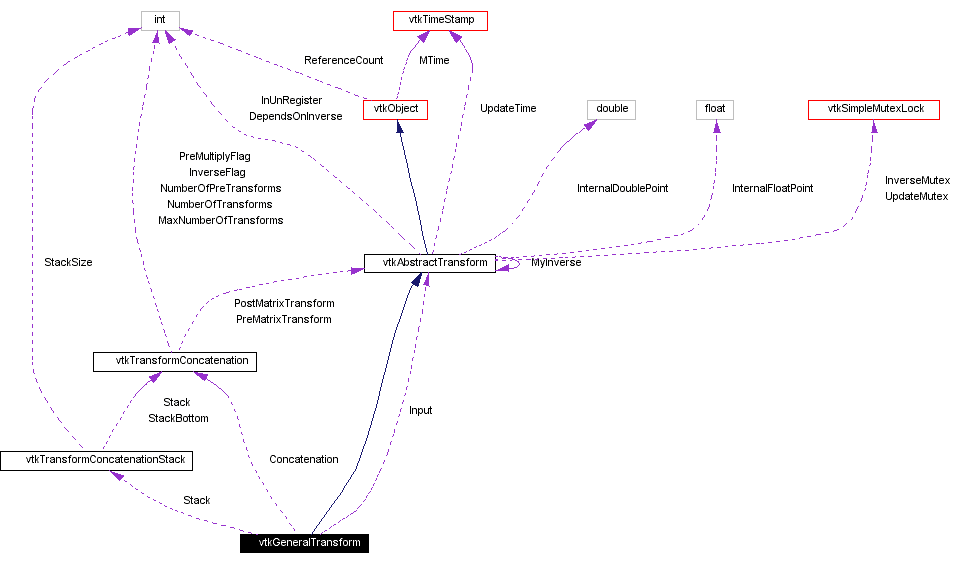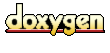Main Page   Class Hierarchy   Alphabetical List   Compound List   File List   Compound Members   File Members   Related Pages

# vtkGeneralTransform Class Reference

allows operations on any transforms. More...

`#include <vtkGeneralTransform.h>`

Inheritance diagram for vtkGeneralTransform:[legend]
Collaboration diagram for vtkGeneralTransform:[legend]
List of all members.

## Public Methods

virtual const char * GetClassName ()
virtual int IsA (const char *type)
void PrintSelf (ostream &os, vtkIndent indent)
void Identity ()
void Inverse ()
void Translate (double x, double y, double z)
void Translate (const double x)
void Translate (const float x)
void RotateWXYZ (double angle, double x, double y, double z)
void RotateWXYZ (double angle, const double axis)
void RotateWXYZ (double angle, const float axis)
void RotateX (double angle)
void RotateY (double angle)
void RotateZ (double angle)
void Scale (double x, double y, double z)
void Scale (const double s)
void Scale (const float s)
void Concatenate (vtkMatrix4x4 *matrix)
void Concatenate (const double elements)
void Concatenate (vtkAbstractTransform *transform)
void PreMultiply ()
void PostMultiply ()
void Push ()
void Pop ()
void SetInput (vtkAbstractTransform *input)
vtkAbstractTransformGetInput ()
void InternalTransformPoint (const float in, float out)
void InternalTransformPoint (const double in, double out)
void InternalTransformDerivative (const float in, float out, float derivative)
void InternalTransformDerivative (const double in, double out, double derivative)
int CircuitCheck (vtkAbstractTransform *transform)
vtkAbstractTransformMakeTransform ()
unsigned long GetMTime ()

## Static Public Methods

vtkGeneralTransform * New ()
int IsTypeOf (const char *type)
vtkGeneralTransform * SafeDownCast (vtkObject *o)

## Protected Methods

vtkGeneralTransform ()
~vtkGeneralTransform ()
vtkGeneralTransform (const vtkGeneralTransform &)
void operator= (const vtkGeneralTransform &)
void InternalDeepCopy (vtkAbstractTransform *t)
void InternalUpdate ()

## Protected Attributes

vtkAbstractTransformInput
vtkTransformConcatenationConcatenation
vtkTransformConcatenationStackStack

## Detailed Description

allows operations on any transforms.

Date:
2000/12/10 20:08:10
Revision:
1.30
Thanks:
Thanks to David G. Gobbi who developed this class.
vtkGeneralTransform is like vtkTransform and vtkPerspectiveTransform, but it will work with any vtkAbstractTransform as input. It is not as efficient as the other two, however, because arbitrary transformations cannot be concatenated by matrix multiplication. Transform concatenation is simulated by passing each input point through each transform in turn.
vtkTransform vtkPerspectiveTransform
Examples:
vtkGeneralTransform (examples)

Definition at line 64 of file vtkGeneralTransform.h.

## Constructor & Destructor Documentation

 vtkGeneralTransform::vtkGeneralTransform ( ) ` [protected]`

 vtkGeneralTransform::~vtkGeneralTransform ( ) ` [protected]`

 vtkGeneralTransform::vtkGeneralTransform ( const vtkGeneralTransform & ) ` [inline, protected]`
 Definition at line 195 of file vtkGeneralTransform.h.

## Member Function Documentation

 vtkGeneralTransform* vtkGeneralTransform::New ( ) ` [static]`
 Create an object with Debug turned off, modified time initialized to zero, and reference counting on. Reimplemented from vtkObject.

 virtual const char* vtkGeneralTransform::GetClassName ( ) ` [virtual]`
 Return the class name as a string. This method is defined in all subclasses of vtkObject with the vtkTypeMacro found in vtkSetGet.h. Reimplemented from vtkAbstractTransform.

 int vtkGeneralTransform::IsTypeOf ( const char * type ) ` [static]`
 Return 1 if this class type is the same type of (or a subclass of) the named class. Returns 0 otherwise. This method works in combination with vtkTypeMacro found in vtkSetGet.h. Reimplemented from vtkAbstractTransform.

 virtual int vtkGeneralTransform::IsA ( const char * type ) ` [virtual]`
 Return 1 if this class is the same type of (or a subclass of) the named class. Returns 0 otherwise. This method works in combination with vtkTypeMacro found in vtkSetGet.h. Reimplemented from vtkAbstractTransform.

 vtkGeneralTransform* vtkGeneralTransform::SafeDownCast ( vtkObject * o ) ` [static]`
 Will cast the supplied object to vtkObject* is this is a safe operation (i.e., a safe downcast); otherwise NULL is returned. This method is defined in all subclasses of vtkObject with the vtkTypeMacro found in vtkSetGet.h. Reimplemented from vtkAbstractTransform.

 void vtkGeneralTransform::PrintSelf ( ostream & os, vtkIndent indent ) ` [virtual]`
 Methods invoked by print to print information about the object including superclasses. Typically not called by the user (use Print() instead) but used in the hierarchical print process to combine the output of several classes. Reimplemented from vtkAbstractTransform.

 void vtkGeneralTransform::Identity ( ) ` [inline]`
 Set this transformation to the identity transformation. If the transform has an Input, then the transformation will be reset so that it is the same as the Input. Reimplemented from vtkAbstractTransform. Definition at line 75 of file vtkGeneralTransform.h.

 void vtkGeneralTransform::Inverse ( ) ` [inline, virtual]`
 Invert the transformation. This will also set a flag so that the transformation will use the inverse of its Input, if an Input has been set. Reimplemented from vtkAbstractTransform. Definition at line 80 of file vtkGeneralTransform.h.

 void vtkGeneralTransform::Translate ( double x, double y, double z ) ` [inline]`
 Create a translation matrix and concatenate it with the current transformation according to PreMultiply or PostMultiply semantics. Definition at line 84 of file vtkGeneralTransform.h.

 void vtkGeneralTransform::Translate ( const double x ) ` [inline]`
 Definition at line 86 of file vtkGeneralTransform.h.

 void vtkGeneralTransform::Translate ( const float x ) ` [inline]`
 Definition at line 87 of file vtkGeneralTransform.h.

 void vtkGeneralTransform::RotateWXYZ ( double angle, double x, double y, double z ) ` [inline]`
 Create a rotation matrix and concatenate it with the current transformation according to PreMultiply or PostMultiply semantics. The angle is in degrees, and (x,y,z) specifies the axis that the rotation will be performed around. Definition at line 93 of file vtkGeneralTransform.h.

 void vtkGeneralTransform::RotateWXYZ ( double angle, const double axis ) ` [inline]`
 Definition at line 95 of file vtkGeneralTransform.h.

 void vtkGeneralTransform::RotateWXYZ ( double angle, const float axis ) ` [inline]`
 Definition at line 97 of file vtkGeneralTransform.h.

 void vtkGeneralTransform::RotateX ( double angle ) ` [inline]`
 Create a rotation matrix about the X, Y, or Z axis and concatenate it with the current transformation according to PreMultiply or PostMultiply semantics. The angle is expressed in degrees. Definition at line 103 of file vtkGeneralTransform.h.

 void vtkGeneralTransform::RotateY ( double angle ) ` [inline]`
 Definition at line 104 of file vtkGeneralTransform.h.

 void vtkGeneralTransform::RotateZ ( double angle ) ` [inline]`
 Definition at line 105 of file vtkGeneralTransform.h.

 void vtkGeneralTransform::Scale ( double x, double y, double z ) ` [inline]`
 Create a scale matrix (i.e. set the diagonal elements to x, y, z) and concatenate it with the current transformation according to PreMultiply or PostMultiply semantics. Definition at line 110 of file vtkGeneralTransform.h.

 void vtkGeneralTransform::Scale ( const double s ) ` [inline]`
 Definition at line 112 of file vtkGeneralTransform.h.

 void vtkGeneralTransform::Scale ( const float s ) ` [inline]`
 Definition at line 113 of file vtkGeneralTransform.h.

 void vtkGeneralTransform::Concatenate ( vtkMatrix4x4 * matrix ) ` [inline]`
 Concatenates the matrix with the current transformation according to PreMultiply or PostMultiply semantics. Definition at line 117 of file vtkGeneralTransform.h.

 void vtkGeneralTransform::Concatenate ( const double elements ) ` [inline]`
 Definition at line 119 of file vtkGeneralTransform.h.

 void vtkGeneralTransform::Concatenate ( vtkAbstractTransform * transform )
 Concatenate the specified transform with the current transformation according to PreMultiply or PostMultiply semantics. The concatenation is pipelined, meaning that if any of the transformations are changed, even after Concatenate() is called, those changes will be reflected when you call TransformPoint().

 void vtkGeneralTransform::PreMultiply ( ) ` [inline]`
 Sets the internal state of the transform to PreMultiply. All subsequent operations will occur before those already represented in the current transformation. In homogeneous matrix notation, M = M*A where M is the current transformation matrix and A is the applied matrix. The default is PreMultiply. Definition at line 134 of file vtkGeneralTransform.h.

 void vtkGeneralTransform::PostMultiply ( ) ` [inline]`
 Sets the internal state of the transform to PostMultiply. All subsequent operations will occur after those already represented in the current transformation. In homogeneous matrix notation, M = A*M where M is the current transformation matrix and A is the applied matrix. The default is PreMultiply. Definition at line 143 of file vtkGeneralTransform.h.

 void vtkGeneralTransform::Push ( ) ` [inline]`
 Pushes the current transformation onto the transformation stack. Definition at line 148 of file vtkGeneralTransform.h.

 void vtkGeneralTransform::Pop ( ) ` [inline]`
 Deletes the transformation on the top of the stack and sets the top to the next transformation on the stack. Definition at line 155 of file vtkGeneralTransform.h.

 void vtkGeneralTransform::SetInput ( vtkAbstractTransform * input )
 Set the input for this transformation. This will be used as the base transformation if it is set. This method allows you to build a transform pipeline: if the input is modified, then this transformation will automatically update accordingly.

 vtkAbstractTransform* vtkGeneralTransform::GetInput ( ) ` [inline]`
 Definition at line 164 of file vtkGeneralTransform.h.

 void vtkGeneralTransform::InternalTransformPoint ( const float in, float out ) ` [virtual]`
 This will calculate the transformation without calling Update. Meant for use only within other VTK classes. Reimplemented from vtkAbstractTransform.

 void vtkGeneralTransform::InternalTransformPoint ( const double in, double out ) ` [virtual]`
 Reimplemented from vtkAbstractTransform.

 void vtkGeneralTransform::InternalTransformDerivative ( const float in, float out, float derivative ) ` [virtual]`
 This will calculate the transformation as well as its derivative without calling Update. Meant for use only within other VTK classes. Reimplemented from vtkAbstractTransform.

 void vtkGeneralTransform::InternalTransformDerivative ( const double in, double out, double derivative ) ` [virtual]`
 Reimplemented from vtkAbstractTransform.

 int vtkGeneralTransform::CircuitCheck ( vtkAbstractTransform * transform ) ` [virtual]`
 Check for self-reference. Will return true if concatenating with the specified transform, setting it to be our inverse, or setting it to be our input will create a circular reference. CircuitCheck is automatically called by SetInput(), SetInverse(), and Concatenate(vtkXTransform *). Avoid using this function, it is experimental. Reimplemented from vtkAbstractTransform.

 vtkAbstractTransform* vtkGeneralTransform::MakeTransform ( ) ` [virtual]`
 Make another transform of the same type. Reimplemented from vtkAbstractTransform.

 unsigned long vtkGeneralTransform::GetMTime ( ) ` [virtual]`
 Override GetMTime to account for input and concatenation. Reimplemented from vtkAbstractTransform.

 void vtkGeneralTransform::operator= ( const vtkGeneralTransform & ) ` [inline, protected]`
 Definition at line 196 of file vtkGeneralTransform.h.

 void vtkGeneralTransform::InternalDeepCopy ( vtkAbstractTransform * t ) ` [protected, virtual]`
 Perform any subclass-specific DeepCopy. Reimplemented from vtkAbstractTransform.

 void vtkGeneralTransform::InternalUpdate ( ) ` [protected, virtual]`
 Perform any subclass-specific Update. Reimplemented from vtkAbstractTransform.

## Member Data Documentation

 vtkAbstractTransform* vtkGeneralTransform::Input` [protected]`
 Definition at line 201 of file vtkGeneralTransform.h.

 vtkTransformConcatenation* vtkGeneralTransform::Concatenation` [protected]`
 Definition at line 202 of file vtkGeneralTransform.h.

 vtkTransformConcatenationStack* vtkGeneralTransform::Stack` [protected]`
 Definition at line 203 of file vtkGeneralTransform.h.

The documentation for this class was generated from the following file:

Generated on Wed Nov 21 12:48:02 2001 for VTK by1.2.11.1 written by Dimitri van Heesch, © 1997-2001